# Intermediate Algebra Tutorial 32

Intermediate Algebra
Tutorial 32: Multiplying and Dividing Rational ExpressionsAnswer/Discussion to 1a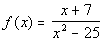Our restriction here is that the denominator of a fraction can never be equal to 0.  So to find our domain, we want to set the denominator "not equal" to 0 to restrict those values.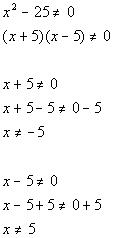*Restrict values that cause den. to be 0

*"Solve" for x

*"Solve" for x

Our domain is all real numbers except -5 and 5 because they both make the denominator equal to 0, which would not give us a real number answer for our function.Answer/Discussion to 2a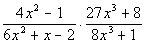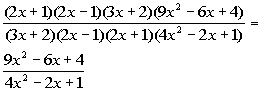*Factor the num. and den.

*Divide out the common factors
of (2x + 1), (2x - 1), and (3x + 2)Answer/Discussion to 2b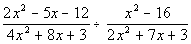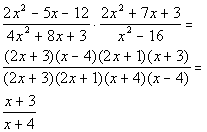*Rewrite as mult. of reciprocal

*Factor the num. and den.

*Div. out the common factors of
(2x + 3), (x - 4), and (2x + 1)Answer/Discussion to 2c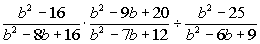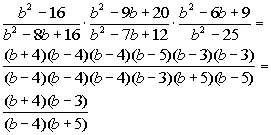*Rewrite the div. as mult. of recip.

*Factor the num. and den.

*Div. out the common factors of
(b - 4), (b - 4), (b - 5), and (b - 3)